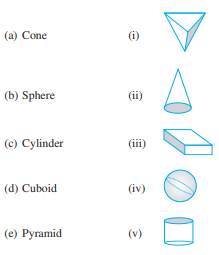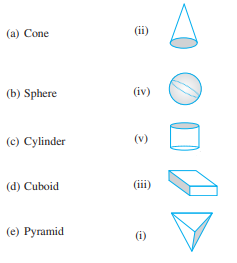## Ex 5.9 Question 1.

Match the following:Give two examples of each shape.
Solution:(a) Examples:
(i) An ice-cream cone
(ii) Birthday cap

(b) Examples:
(i) Tennis ball
(ii) Cricket ball

(c) Examples:
(ii) A lawn roller

(d) Examples:
(i) Math book
(ii) A brick

(e) Examples:
(i) A diamond
(ii) Egypt-Pyramids

What shape is
(b) A brick?
(b) A matchbox?